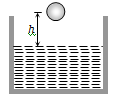#28 | Solved Problems: Set 6
(Physics) > Mechanical Properties of Fluids
Related Practice Questions :

Spherical balls of radius 'r' are falling in a viscous fluid of viscosity '$\mathrm{\eta }$' with a velocity 'v'. The retarding viscous force acting on the spherical ball is

(a) Inversely proportional to 'r' but directly proportional to velocity 'v'

(b) Directly proportional to both radius 'r' and velocity 'v'

(c) Inversely proportional to both radius 'r' and velocity 'v'

(d) Directly proportional to 'r' but inversely proportional to 'v'

Complete Question Bank + Test Series
Complete Question Bank

Difficulty Level:

A small sphere of mass m is dropped from a great height. After it has fallen 100 m, it has attained its terminal velocity and continues to fall at that speed. The work done by air friction against the sphere during the first 100 m of fall is

(a) Greater than the work done by air friction in the second 100 m

(b) Less than the work done by air friction in the second 100 m

(c) Equal to 100 mg

(d) Greater than 100 mg

Complete Question Bank + Test Series
Complete Question Bank

Difficulty Level:

A ball of radius r and density $\mathrm{\rho }$ falls freely under gravity through a distance h before entering water. Velocity of ball does not change even on entering water. If viscosity of water is $\mathrm{\eta }$, the value of h is given by(a) $\frac{2}{9}{\mathrm{r}}^{2}\left(\frac{1-\mathrm{\rho }}{\mathrm{\eta }}\right)\mathrm{g}$

(b) $\frac{2}{81}{\mathrm{r}}^{2}\left(\frac{\mathrm{\rho }-1}{\mathrm{\eta }}\right)\mathrm{g}$

(c) $\frac{2}{81}{\mathrm{r}}^{4}{\left(\frac{\mathrm{\rho }-1}{\mathrm{\eta }}\right)}^{2}\mathrm{g}$

(d) $\frac{2}{9}{\mathrm{r}}^{4}{\left(\frac{\mathrm{\rho }-1}{\mathrm{\eta }}\right)}^{2}\mathrm{g}$

Complete Question Bank + Test Series
Complete Question Bank

Difficulty Level:

The rate of steady volume flow of water through a capillary tube of length 'l' and radius 'r' under a pressure difference of P is V. This tube is connected with another tube of the same length but half the radius in series. Then the rate of steady volume flow through them is (The pressure difference across the combination is P)

(a) $\frac{\mathrm{V}}{16}$                                     (b) $\frac{\mathrm{V}}{17}$

(c) $\frac{16\mathrm{V}}{17}$                                    (d) $\frac{17\mathrm{V}}{16}$

Complete Question Bank + Test Series
Complete Question Bank

Difficulty Level:

A liquid is flowing in a horizontal uniform capillary tube under a constant pressure difference P. The value of pressure for which the rate of flow of the liquid is doubled when the radius and length both are doubled is

(a) P                             (b) $\frac{3\mathrm{P}}{4}$

(c) $\frac{\mathrm{P}}{2}$                           (d) $\frac{\mathrm{P}}{4}$